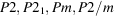International Tables for Crystallography (2006). Vol. A, ch. 15.2, pp. 879-899https://doi.org/10.1107/97809553602060000534

## Contents

• 15.2. Euclidean and affine normalizers of plane groups and space groups  (pp. 879-899)
• 15.2.1. Euclidean normalizers of plane groups and space groups  (pp. 879-882) | html | pdf |
• 15.2.2. Affine normalizers of plane groups and space groups  (pp. 882-894) | html | pdf |
• References | html | pdf |
• Figures
• Fig. 15.2.1.1. Parameter range for space groups of typesand(plane groups of types p 1 and p 2)  (p. 879) | html | pdf |
• Fig. 15.2.1.2. Parameter range for space groups of types C 2, Pc , Cm , Cc ,,,and(p. 879) | html | pdf |
• Fig. 15.2.1.3. Parameter range for space groups of types C 2, Pc , Cm , Cc ,,,and(p. 880) | html | pdf |
• Fig. 15.2.1.4. Parameter range for space groups of types C 2, Pc , Cm , Cc ,,,and(p. 880) | html | pdf |
• Tables
• Table 15.2.1.1. Euclidean normalizers of the plane groups  (p. 881) | html | pdf |
• Table 15.2.1.2. Euclidean normalizers of the triclinic space groups  (p. 882) | html | pdf |
• Table 15.2.1.3. Euclidean normalizers of the monoclinic and orthorhombic space groups  (pp. 883-894) | html | pdf |
• Table 15.2.1.4. Euclidean normalizers of the tetragonal, trigonal, hexagonal and cubic space groups  (pp. 895-898) | html | pdf |
• Table 15.2.2.1. Affine normalizers of the triclinic and monoclinic space groups  (p. 899) | html | pdf |
• Table 15.2.2.2. Matrices and vectors used in Table 15.2.2.1 for the description of the affine normalizers of monoclinic and triclinic space groups  (p. 899) | html | pdf |